# Data Manipulation in Excel Cheat Sheet

Discover how to manipulate data in Excel using this convenient cheat sheet!
May 2023  · 4 min read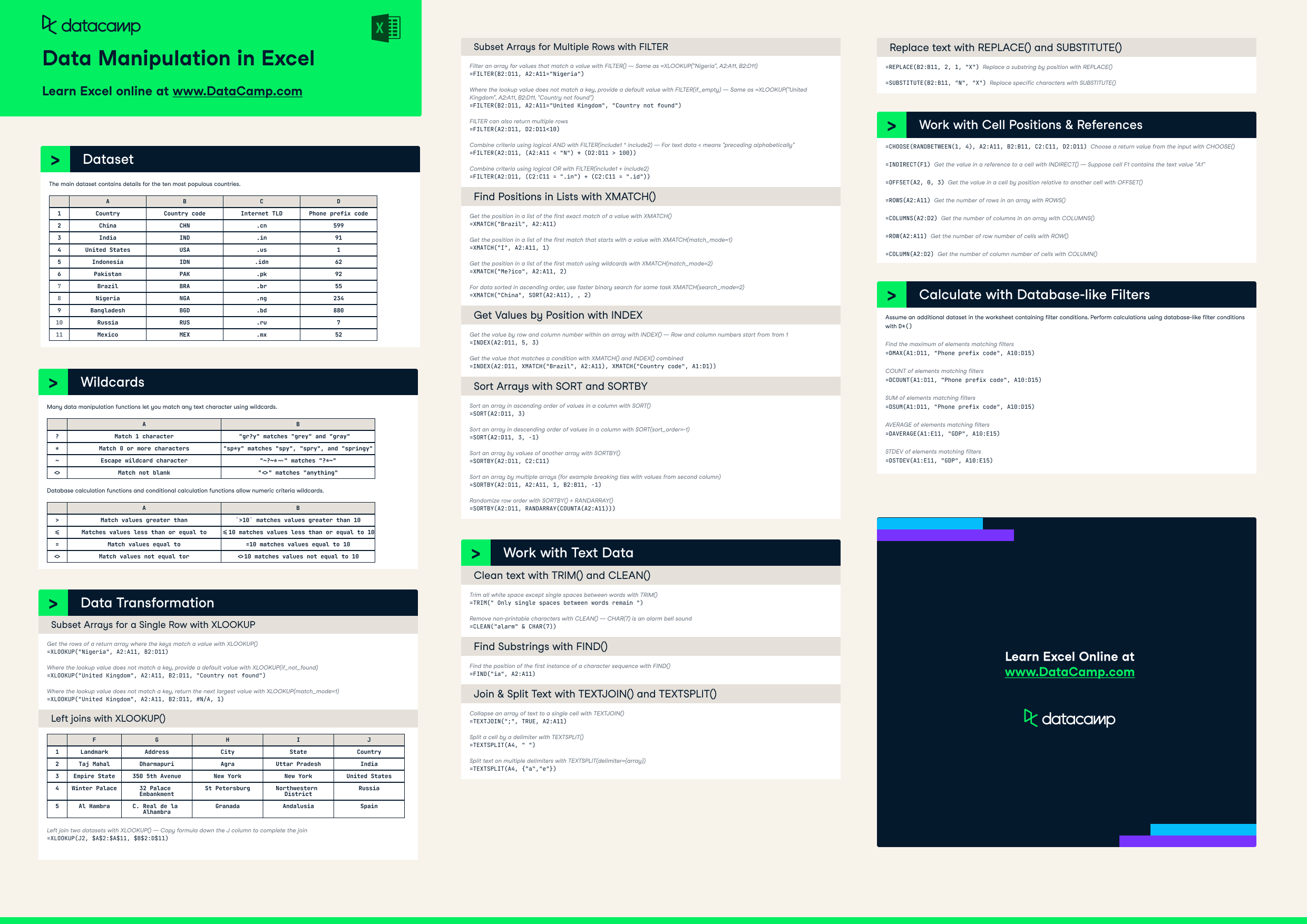Have this cheat sheet at your fingertips

## Dataset

The main dataset contains details for the ten most populous countries.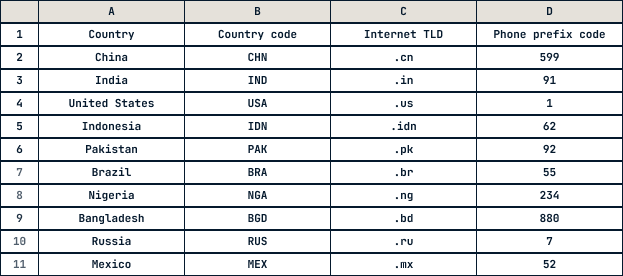## Wildcards

Many data manipulation functions let you match any text character using wildcards.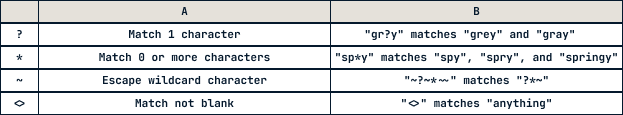Database calculation functions and conditional calculation functions allow numeric criteria wildcards.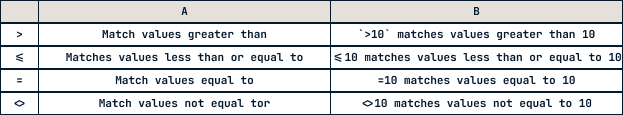## Data Transformation

### Subset Arrays for a Single Row with XLOOKUP

Get the rows of a return array where the keys match a value with XLOOKUP()

`=XLOOKUP("Nigeria", A2:A11, B2:D11) `

Where the lookup value does not match a key, provide a default value with XLOOKUP(if_not_found)

`=XLOOKUP("United Kingdom", A2:A11, B2:D11, "Country not found") `

Where the lookup value does not match a key, return the next largest value with XLOOKUP(match_mode=1)

`=XLOOKUP("United Kingdom", A2:A11, B2:D11, #N/A, 1)`

### Left joins with XLOOKUP()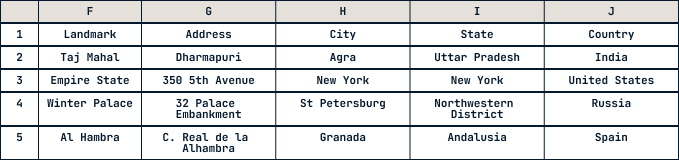Left join two datasets with XLOOKUP() — Copy formula down the J column to complete the join

`=XLOOKUP(J2, \$A\$2:\$A\$11, \$B\$2:D\$11)`

### Subset Arrays for Multiple Rows with FILTER

Filter an array for values that match a value with FILTER() — Same as

`=XLOOKUP("Nigeria", A2:A11, B2:D11)=FILTER(B2:D11, A2:A11="Nigeria") `

Where the lookup value does not match a key, provide a default value with FILTER(if_empty) — Same as  =XLOOKUP("United Kingdom", A2:A11, B2:D11, "Country not found")

`=FILTER(B2:D11, A2:A11="United Kingdom", "Country not found") `

FILTER can also return multiple rows

`=FILTER(A2:D11, D2:D11<10) `

Combine criteria using logical AND with FILTER(include1 * include2) — For text data < means "preceding alphabetically"

`=FILTER(A2:D11, (A2:A11 < "N") * (D2:D11 > 100)) `

Combine criteria using logical OR with FILTER(include1 + include2)

`=FILTER(A2:D11, (C2:C11 = ".in") + (C2:C11 = ".id"))`

### Find Positions in Lists with XMATCH()

Get the position in a list of the first exact match of a value with XMATCH()

`=XMATCH("Brazil", A2:A11) `

Get the position in a list of the first match that starts with a value with XMATCH(match_mode=1)

`=XMATCH("I", A2:A11, 1) `

Get the position in a list of the first match using wildcards with XMATCH(match_mode=2)

`=XMATCH("Me?ico", A2:A11, 2) `

For data sorted in ascending order, use faster binary search for same task XMATCH(search_mode=2)

`=XMATCH("China", SORT(A2:A11), , 2)`

### Get Values by Position with INDEX

Get the value by row and column number within an array with INDEX() — Row and column numbers start from 1rom 1

`=INDEX(A2:D11, 5, 3) `

Get the value that matches a condition with XMATCH() and INDEX() combined

`=INDEX(A2:D11, XMATCH("Brazil", A2:A11), XMATCH("Country code", A1:D1))`

### Sort Arrays with SORT and SORTBY

Sort an array in ascending order of values in a column with SORT()

`=SORT(A2:D11, 3) `

Sort an array in descending order of values in a column with SORT(sort_order=-1)

`=SORT(A2:D11, 3, -1)`

Sort an array by values of another array with SORTBY()

`=SORTBY(A2:D11, C2:C11) `

Sort an array by multiple arrays (for example breaking ties with values from second column)

`=SORTBY(A2:D11, A2:A11, 1, B2:B11, -1) `

Randomize row order with SORTBY() + RANDARRAY()

`=SORTBY(A2:D11, RANDARRAY(COUNTA(A2:A11)))`

## Work with Text Data

### Clean text with TRIM() and CLEAN()

Trim all white space except single spaces between words with TRIM()

`=TRIM(" Only single spaces between words remain ") `

Remove non-printable characters with CLEAN() — CHAR(7) is an alarm bell sound

`=CLEAN("alarm" & CHAR(7))`

### Find Substrings with FIND()

Find the position of the first instance of a character sequence with FIND()

`=FIND("ia", A2:A11)`

### Join & Split Text with TEXTJOIN() and TEXTSPLIT()

Collapse an array of text to a single cell with TEXTJOIN()

`=TEXTJOIN(";", TRUE, A2:A11) `

Split a cell by a delimiter with TEXTSPLIT()

`=TEXTSPLIT(A4, " ") `

Split text on multiple delimiters with TEXTSPLIT(delimiter={array})

`=TEXTSPLIT(A4, {"a","e"})`

### Replace text with REPLACE() and SUBSTITUTE()

`=REPLACE(B2:B11, 2, 1, "X")` Replace a substring by position with REPLACE()

`=SUBSTITUTE(B2:B11, "N", "X")` Replace specific characters with SUBSTITUTE()

## Work with Cell Positions & References

`=CHOOSE(RANDBETWEEN(1, 4), A2:A11, B2:B11, C2:C11, D2:D11)` Choose a return value from the input with CHOOSE()

`=INDIRECT(F1)` Get the value in a reference to a cell with INDIRECT() — Suppose cell F1 contains the text value "A1"

`=OFFSET(A2, 0, 3)` Get the value in a cell by position relative to another cell with OFFSET()

`=ROWS(A2:A11)` Get the number of rows in an array with ROWS()

`=COLUMNS(A2:D2)` Get the number of columns in an array with COLUMNS()

`=ROW(A2:A11)` Get the number of row number of cells with ROW()

`=COLUMN(A2:D2)` Get the number of column number of cells with COLUMN()

## Calculate with Database-like Filters

Assume an additional dataset in the worksheet containing filter conditions. Perform calculations using database-like filter conditions with D*()

Find the maximum of elements matching filters

`=DMAX(A1:D11, "Phone prefix code", A10:D15)`

COUNT of elements matching filters

`=DCOUNT(A1:D11, "Phone prefix code", A10:D15)`

SUM of elements matching filters

`=DSUM(A1:D11, "Phone prefix code", A10:D15)`

AVERAGE of elements matching filters

`=DAVERAGE(A1:E11, "GDP", A10:E15)`

STDEV of elements matching filters

`=DSTDEV(A1:E11, "GDP", A10:E15)`

## Master your data skills with DataCamp

More than 10 million people learn Python, R, SQL, and other tech skills using our hands-on courses crafted by industry experts.Related

### The Ultimate List of Data Science Podcasts to Listen to Right Now

We’ve hand-picked the ultimate list of top data science podcasts for you to gain a deeper understanding of data science and analytics. Add them to your playlist now!

Shaun Edmond

8 min

### Excel Basics Cheat Sheet

Learn the basics of Excel with our quick and easy cheat sheet. Have the basics of formulas, operators, math functions and more at your fingertips.

### Time Series Analysis with Spreadsheets Tutorial

In this tutorial, you'll learn basic time-series concepts and basic methods for forecasting time series data using spreadsheets.Avinash Navlani

9 min

### The 15 Basic Excel Formulas Everyone Needs to Know

Learn how to add arithmetic, string, time series, and complex formulas in Microsoft Excel.

### How to Create a Dashboard in Excel in 3 Easy Steps

Learn everything you need to know about how to create a dashboard in Excel, with tips and examples.

### How to Analyze Data in Google Sheets With Python: A Step-By-Step Guide

Boost your data analysis skills with our step-by-step guide on how to analyze, manipulate and write back data in Google Sheets using Python.
See MoreSee More